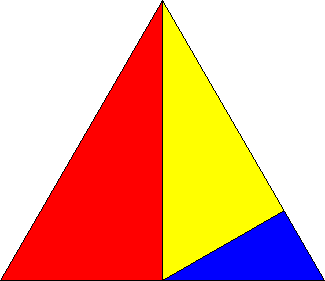# Puzzle of the Week for 1 February 1999: Solution

A square divided into three congruent pieces: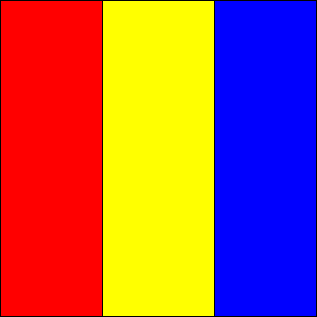A square divided into three similar pieces, two of which are congruent: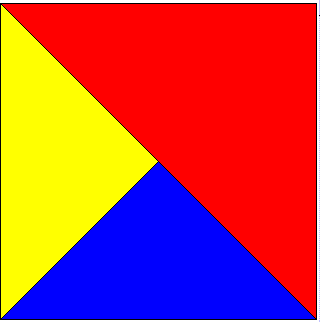There is another solution, which yields rectangular pieces. Are there others?

A square divided into three similar pieces, none of which are congruent: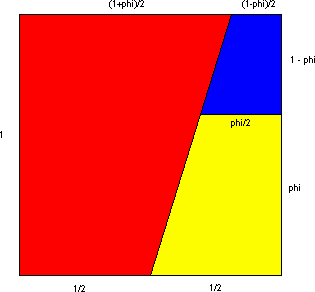(In this dissection, phi is the "golden ratio", 0.61803....)

A triangle divided into three congruent pieces: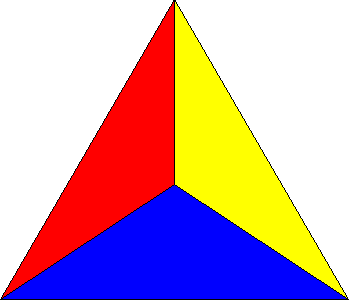There are many variations on this solution.

A triangle divided into three similar pieces, two of which are congruent: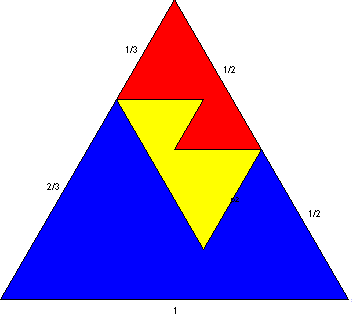Is this solution unique?

A triangle divided into three similar pieces, none of which are congruent: